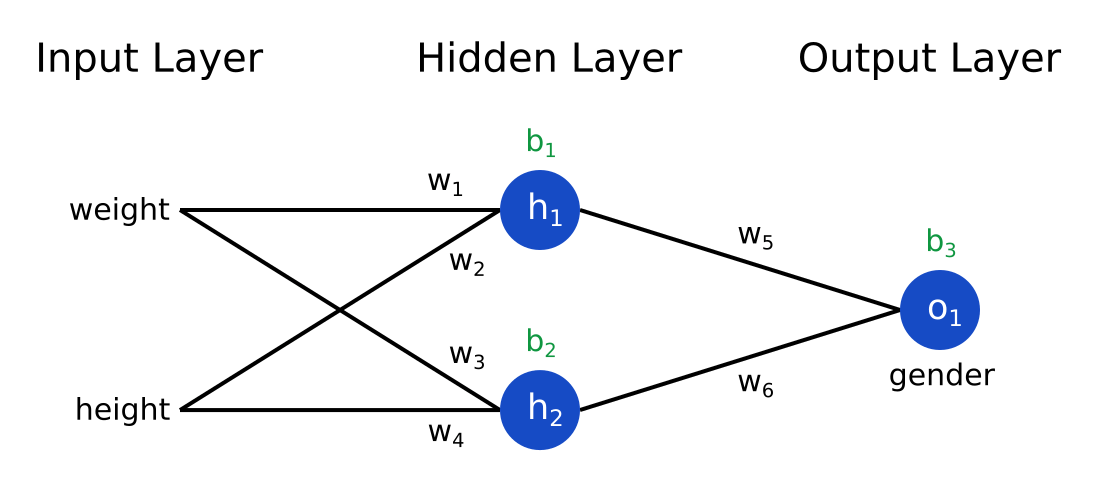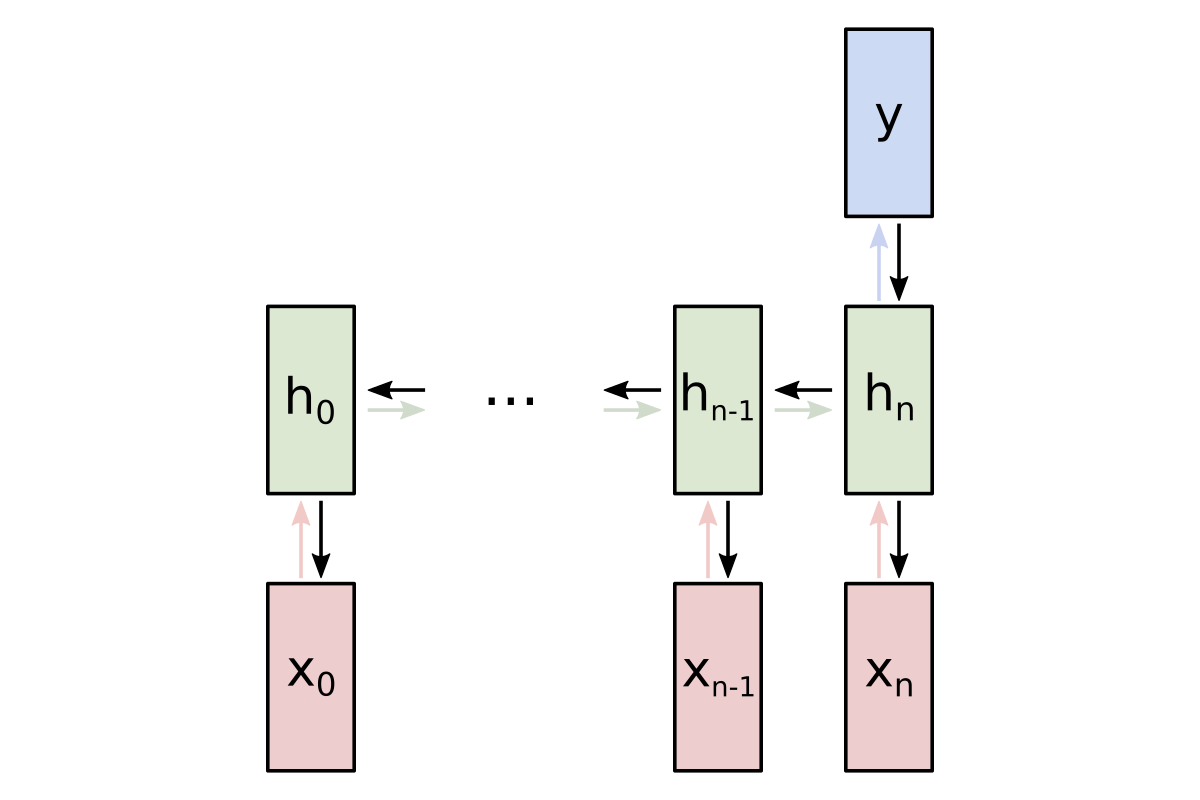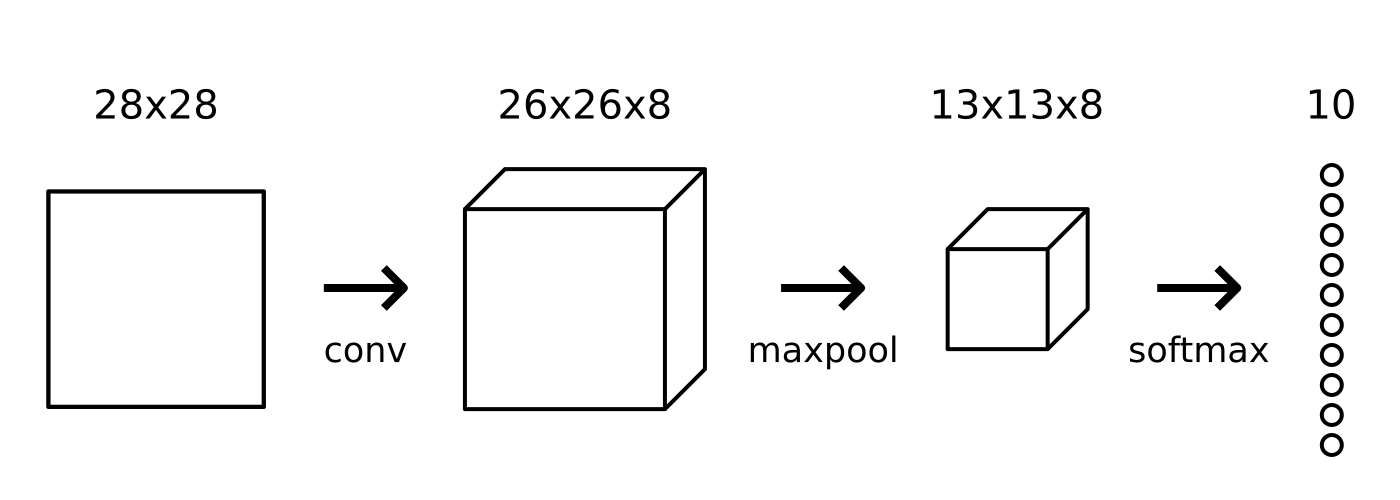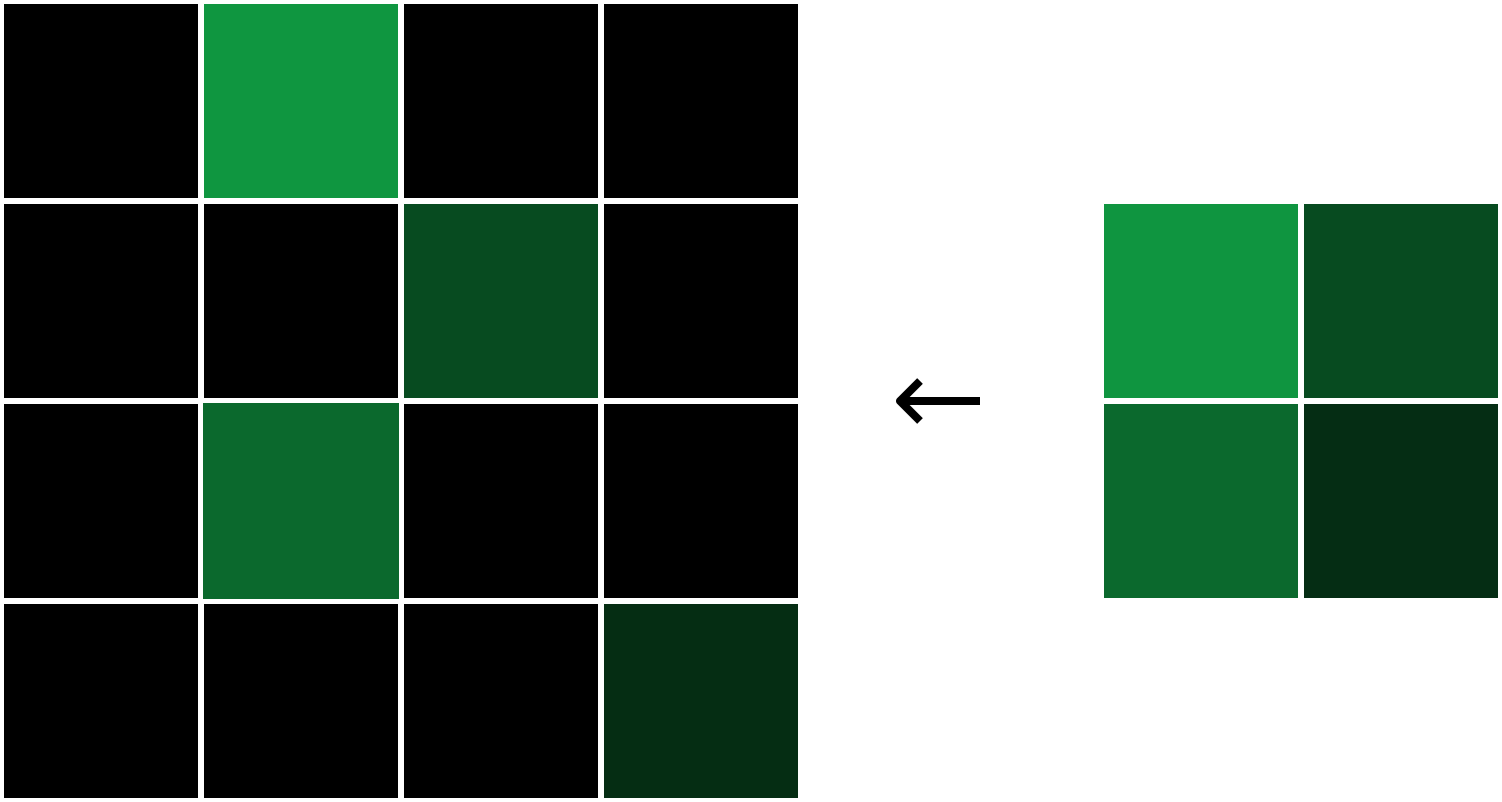# Neural Networks From ScratchThis 4-post series, written especially with beginners in mind, provides a fundamentals-oriented approach towards understanding Neural Networks. We’ll start with an introduction to classic Neural Networks for complete beginners before delving into two popular variants: Recurrent Neural Networks (RNNs) and Convolutional Neural Networks (CNNs).

For each of each these types of networks, we’ll:

• See the structure of the network.
• Understand the motivation behind using that type of network.
• Introduce a real-world problem that can be solved using that network.
• Manually derive the gradients needed to train our problem-specific network.
• Implement a fully-functioning network completely from scratch (using only numpy) in Python.

## Background

This series requires ZERO prior knowledge of Machine Learning or Neural Networks. However, background in the following topics may be helpful:

• Multivariable Calculus, used when deriving the gradients needed to train our networks. These gradient derivations can be skipped if you don’t have the background.
• Linear Algebra, specifically Matrix algebra - matrices are often the best way to represent weights for Neural Networks.
• Python 3, because the Python implementations in these posts are a major part of their educational value. A baseline proficiency in Python is enough.

## The Series

Ready to get started? Here we go:### 1. Machine Learning for Beginners: An Introduction to Neural Networks

A simple explanation of how they work and how to implement one from scratch in Python.### 2. An Introduction to Recurrent Neural Networks for Beginners

A simple walkthrough of what RNNs are, how they work, and how to build one from scratch in Python.### 3. CNNs, Part 1: An Introduction to Convolutional Neural Networks

A simple guide to what CNNs are, how they work, and how to build one from scratch in Python.### 4. CNNs, Part 2: Training a Convolutional Neural Network

A simple walkthrough of deriving backpropagation for CNNs and implementing it from scratch in Python.

## What Next?

Still eager to learn? Some more things you can do include:

I plan on writing more about Neural Networks in the future, so subscribe to my newsletter if you want to get notified of new content.